## Controlling The Real World With Computers

 Home Order Let me know what you think

1) B) Schematic

2) D) Switch

3) A) Resistor

4) C) Ohm, Ohm's Law

5) A) Amperes (or Amps), Milliamps (or Ma or ma)

6) C) Capacitors

7) B) Diode

8) D) Integrated Circuits

9) B) Operational Amplifier

10) This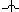is unconnected conductors.

11) This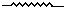is a resistor.

12) This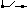is a switch.

13) This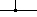is connected conductors.

14) Thisis a capacitor.

15) This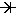is a diode.

16) This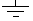is the symbol for ground.

17) Ohm's Law: V = IR

18) I = 4, R = 10 so V = IR = 40 Volts

19) V = 12, R = 6 so I = V/R = 2 Amps

20) I = 75, V = 150K Volts so R = 150K/75 = 2K Ohms